Tamilnadu State Board New Syllabus Samacheer Kalvi 12th Maths Guide Pdf Chapter 6 Applications of Vector Algebra Ex 6.9 Textbook Questions and Answers, Notes.

## Tamilnadu Samacheer Kalvi 12th Maths Solutions Chapter 6 Applications of Vector Algebra Ex 6.9

Question 1.
Find the equation of the plane passing through the line of intersection of the planes $$\overline { r }$$ = (2$$\hat { i }$$ – 7$$\hat { j }$$ + 4$$\hat { k }$$) = 3 and 3x – 5y + 4z + 11 = 0 and the point (- 2, 1, 3).
Solution:
Given planes are
$$\vec{r} \cdot(2 \hat{i}-7 \hat{j}+4 \hat{k})$$ = 3
2x – 7y + 4z – 3 = 0 and 3x – 5y + 4z + 11 = 0
Equation of a plane which passes through the line of intersection of the planes
(2x – 7y + 4z – 3) + λ(3x – 5y + 4z + 11) = 0 …………… (1)
This passes through the point (-2, 1, 3).
(1) ⇒ (-4 – 7 + 12 – 3) + λ(-6 – 5 + 12 + 11) = 0
-2 + λ(12) = 0 ⇒ 12λ = 2
λ = $$\frac{2}{12}$$ ⇒ λ = $$\frac{1}{6}$$
The required equation is
(1) ⇒ (2x – 7y + 4z – 3) + $$\frac{1}{6}$$ (3x – 5y + 4z + 11) = 0
12x – 42y + 24z – 18 + 3x – 5y + 4z + 11 = 0
15x – 47y + 28z – 7 = 0Question 2.
Find the equation of the plane passing through the line of intersection of the planes x + 2y + 3z = 2 and x – y + z = 3 and at a distance $$\frac { 2 }{ √3 }$$ from the point (3, 1, -1).
Solution:
Required equation of the plane
(x + 2y + 3z – 2) + λ(x – y + z – 3) = 0 ………. (1)
(1 + λ)x + (2 – λ)y + (3 + λ)z + (-2 – 3λ) = 0 ………(2)
Distance from (2) to the point (3, 1, -1) is $$\frac { 2 }{ √3 }$$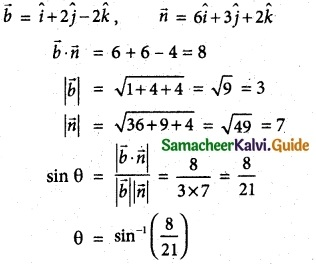putting
λ = $$\frac { -7 }{ 2 }$$ in (1)
The required equation
(x + 2y + 3z – 2) – $$\frac { 7 }{ 2 }$$ (x – y + z – 3) = 0
2x + 4y + 6z – 4 – 7x + 7y – 7z + 21 = 0
-5x + 11y – z + 17 = 0
5x – 11y + z – 17 = 0

Question 3.
Find the angle between the line
$$\overline { r }$$ = (2$$\hat { i }$$ – $$\hat { j }$$ + $$\hat { k }$$) + ($$\hat { i }$$ + 2$$\hat { j }$$ – 2$$\hat { k }$$) and the plane $$\overline { r }$$ (6$$\hat { i }$$ + 3$$\hat { j }$$ + 2$$\hat { k }$$) = 8
Solution: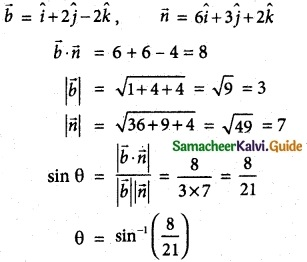Question 4.
Find the angle between the planes $$\overline { r }$$ ($$\hat { i }$$ + $$\hat { j }$$ – 2$$\hat { k }$$) = 3 and 2x – 2y + z = 2.
Solution: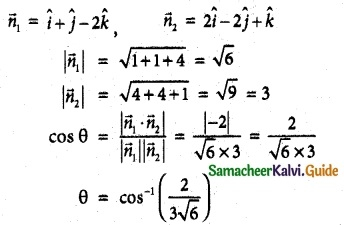Question 5.
Find the equation of the plane which passes through the point (3, 4, -1) and is parallel to the plane 2x – 3y + 5z + 7 = 0. Also, find the distance between the two planes.
Solution:
The required equation parallel to the plane
2x – 3y + 5z + 7 = 0 ………….. (1)
2x – 3y + 5z + λ = 0 ………….. (2)
This passes through (3, 4, -1)
(2) ⇒ 2(3) – 3(4) + 5(-1) + λ = 0
6 – 12 – 5 + 1 = 0
λ = 11
(2) ⇒ The required equation is 2x – 3y + 5z + 11 =0 …………… (3)
∴ Now, distance between the above parallel lines (1) and (3)
a = 2, b = -3, c = 5, d1 = 7, d2 = 11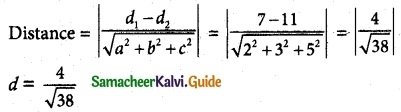Question 6.
Find the length of the perpendicular from the point (1, -2, 3) to the plane x – y + z = 5.
Solution: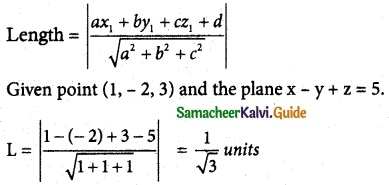Question 7.
Find the point of intersection of the line with the plane (x – 1) = $$\frac { y }{ 2 }$$ = z + 1 with the plane 2x – y – 2z = 2. Also, the angle between the line and the plane.
Solution:
Any point on the line x – 1 = $$\frac{y}{2}$$ = z + 1 is
x – 1 = $$\frac{y}{2}$$ = z + 1 = λ,(say)
(λ + 1, 2λ, λ – 1)
This passes through the plane 2x – y + 2z = 2
2(λ + 1) – 2λ + 2(λ – 1) = 2
2λ + 2 – 2λ + 2λ – 2 = 2
λ = 1
∴ The required point of intersection is (2, 2, 0)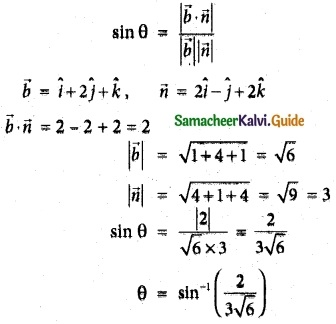Question 8.
Find the co-ordinates of the foot of the perpendicular and length of the perpendicular from the point (4, 3, 2) to the plane x + 2y + 3z = 2.
Solution:
Let us take the point P(4, 3, 2) and Q(x1, y1, z1)
⇒ (x1 – 4, y1 – 3, z1 – 2)
Plane x + 2y + 3z = 2 ………. (1)
Cartesian equation of PQ, $$\frac { x_1-4 }{ 1 }$$ = $$\frac { y_1-3 }{ 2 }$$ = $$\frac { z_3-2 }{ 3 }$$ = λ
(λ + 4, 2λ + 3, 3λ + 2) lies in (1)
(λ + 4) + 2(2λ + 3) + 3(3λ + 2) – 2 = 0
λ + 4 + 4λ + 6 + 9λ + 6 – 2 = 0
14λ + 14 = 0
14λ = -14 .
λ = -1
Co-ordinates of the foot of the ⊥r is (3, 1, -1).
Distance PQ = $$\sqrt{(4-3)^2 + (3-1)^2 + (2+1)^2}$$
= $$\sqrt{1^2 + 2^2 + 3^2}$$ = $$\sqrt{1 + 4 + 9}$$
= $$\sqrt{14}$$ units.